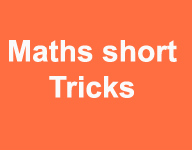# Short trick for simplification calculation questionsNumber of students is preparation the bank exam clerk PO SSC CGL CAT MAT and other competitive exam so simplification topic is very import in all competitive exams. Because number of question put in this topic and calculation used in also other subjects so now we are discussion for short cut and short trick for solve the calculation related question with video in Hindi and English also

1 Question Without detail

54789 – 8462 + 282 – 742 =?

Daily Visit GK on myshort.in

9 – 2 + 2 -2 = 7    (takes the last digit right to left)

8 – 6 + 8 – 4 = 6

7 – 4 + 2 – 7 = -2  Answer is 8    (10 – 2 = 8) (10 nearest number of  2  in the form of 2 digit value )

carry 1      4 – 1 =3    (54789)

3 – 8 = -5 (10 – 5 = 5)

5 – 1 = 4          used  carry 1 rule  (54789)

carry 1 rule : carry the 1 digit to next digit

For example in  case  7 – 4 + 2 – 7 = -2 Answer (  in case 1 carry 4 to 5  that is next digit 54789  )

10 – 2 = 8

4 – 1 = 3 in next step used the 3 not 4

With detail

54789 – 8462 + 282 – 742 =?

1. Step first takes the last digit right to left of the all values (54789 = 9)

9 – 2 + 2 -2 = 7    (9 + 2 -2 -2 = 11 – 4 = 7)

1. Step In second step takes second last digit right to left of the all values (54789 = 8)

8 – 6 + 8 – 4 = 6 (8 + 8 – 6 – 4 = 6)

1. Step same takes next digit right to left (54789 = 7 )

7 – 4 + 2 – 7 = 5 – 7 = -2 in case Answer is 8 (10 – 2 = 8)    (Now in case -2 (remaining – ) so used the 2 digit          nearest number like that in case 10 so used 10 – 2  = 8 and carry 1 number to next digit

(  4 – 1= 3  )     54789

1. Step (54789 = 4 ) (4- 1 = 3) used due to takes 1 carry (54789)

3 – 8 = -5 (10 – 5 = 5) same case 3rd step

Step 5

5 – 1 = 4 (used due to takes 1 carry )

Question : 45.89-2.769+142.8=?

So convert in same form and add extra zero

45.890-002.769+142.800 or write in this type

045.890 – 002.769+142.800

0-9+0 =   used carry rule 10-9-0=1

9-1-6+0=2

8-7+8 = 1+ 8 = 9

5-2+2=5

4-0+4=8

0-0+1=1

185.921

Example 2 =  245 + 412 -121 =?

245 + 412 -121 =?

5 + 2 -1 = 6

4 + 1 – 2 = 3

2+4-1=5

Buy online Rajasthan gk book Railway JE CBT REET PAtwari Book SSC CGL Clerk GD Book Buy online Rajasthan gk book Railway JE CBT REET PAtwari Book SSC CGL Clerk GD Book